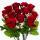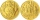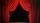Pocket

Mirka spent on a trip for gifts half pocket. For the third remain money bought a bagel. Six euros left her. How many euros had Mirka in pocket?

Result

x =  18

Solution:

x/2+(x-x/2)/3+6 = x

2x = 36

x = 18

Calculated by our simple equation calculator.

Leave us a comment of this math problem and its solution (i.e. if it is still somewhat unclear...):Be the first to comment!To solve this verbal math problem are needed these knowledge from mathematics:

Need help calculate sum, simplify or multiply fractions? Try our fraction calculator. Do you have a linear equation or system of equations and looking for its solution? Or do you have quadratic equation?

Next similar math problems:

1. FactoryIn the factory workers work in three shifts. In the first inning operates half of all employees in the second inning and a third in the third inning 200 employees. How many employees work at the factory?
2. Grandmother and grandfatherGrandmother baked cakes. Grandfather ate half, then quarter of the rest ate Peter and Paul ate half of rest. For parents left 6 cakes. How many cakes maked the grandmother?
3. One thirdIf 3/5 is 360, how much is 1/3?
4. 7 rosesPeter buys 7 roses. When he pay for it left him 4 euro. If he bought 5 roses left him 40 euros. How many euros had Peter before buying?
5. Far countryIn a country far away, the value of 3 pesos is 12 centavos more than the value of 1 peso. How many centavos is 1 peso worth?
6. PerformanceSame number of tickets sold for 15 CZK, 20 CZK, 30 CZK and 40 CZK a total of CZK 13,230 on theatre performance. How many tickets were sold for this performance?
7. FractionsThree-quarters of an unknown number are 4/5. What is 5/6 of this unknown number?
8. Mixed2improperWrite the mixed number as an improper fraction. 166 2/3
9. Simple equation 6Solve equation with one variable: X/2+X/3+X/4=X+4
10. EquationSolve the equation: 1/2-2/8 = 1/10; Write the result as a decimal number.
11. Unknown numberIdentify unknown number which 1/5 is 40 greater than one tenth of that number.
12. Fraction + eqSolve following simple equation with fractions: -5/6(8+5b) = 75 + 5/3b
13. Unknown number 6Determine the unknown number, which is by 1.5 greater than its fourth.
14. Fraction to decimalWrite the fraction 3/22 as a decimal.
15. PipeSteel pipe has a length 2.5 meters. About how many decimetres is 1/3 less than 4/8 of this steel pipe?
16. Equation 20In given equation: 8/9-4/5=2/9+x, find x
17. EqnSolve equation with fractions: 2x/3-50=40+x/4